# Benjamin Montet

The "Random Transiter" has gotten a lot of attention this week since it was posted on the arXiv. And for good reason! It's a really interesting system. Recently, Hugh Osborn tweeted one idea on how to produce transit patterns like this in the case of a brown dwarf binary passing in front of a K+K binary. I wanted to dive in to the system to see if there's anything that was missed in the original analysis. Let's see what we can find!

I'm not going to come to any definitive answers, this is partly me thinking out loud, and partly trying to provoke discussion. If this inspires you to any ideas, let's talk!

In :
```from astropy.io import fits
import numpy as np
import matplotlib.pyplot as plt
```

Let's write down exactly when the dips happen so we can reference them later.

In :
```times = np.array([3161.6826,
3163.3811,
3164.7902,
3165.6969,
3166.0106,
3166.3137,
3174.3337,
3175.3532,
3178.0673,
3181.4968,
3186.5145,
3190.5272,
3195.8316,
3196.8193,
3202.4718,
3202.9034,
3205.6068,
3209.7655,
3216.4965,
3221.1631,
3223.6633,
3225.7971,
3226.5548,
3231.0569,
3238.5381,
3240.6550,
3240.8371,
3243.5688])
```

Multiple teams have made light curves for this star, and there is also the Kepler project's TPF for this object. We might consider each of them in turn, so let's open them up.

In :
```a = fits.open('hlsp_everest_k2_llc_249706694-c15_kepler_v2.0_lc.fits')
tpf = fits.open('ktwo249706694-c15_lpd-targ.fits')
b = fits.open('hlsp_k2sff_k2_lightcurve_249706694-c15_kepler_v1_llc.fits')
```

Let's look at the EVEREST light curve first.

In :
```plt.figure(figsize=(15,5))

plt.plot(a.data['time'], a.data['flux'], '.')
plt.xlabel('Time (BJD-2457000)')
plt.ylabel('Flux')
plt.show()
```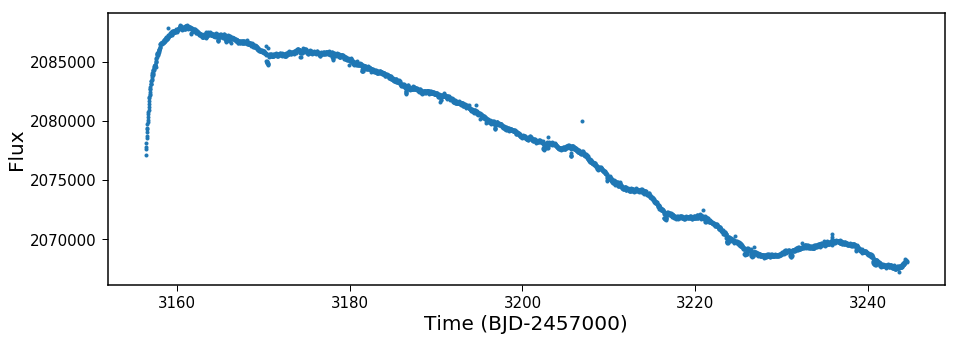There's long term variability, but some of the small dips already stick out. Let's remove the long-term trend and see what it looks like on shorter timescales:

In :
```from lightkurve.lightcurve import LightCurve as LC

plt.figure(figsize=(15,5))
qual  = a.data['quality']
q = qual == 0

lk = LC(a.data['time'][q], a.data['flux'][q])

lkf = lk.flatten(window_length=201).remove_outliers()
plt.plot(lkf.time[100:], lkf.flux[100:], '.')

plt.xlabel('Time (BJD-2457000)')
plt.ylabel('Normalized Flux')

plt.show()

lkp = lkf.to_periodogram()

lkp.plot(format='period', scale='log')
peak = 1/lkp.frequency_at_max_power.value
```
```/Users/ozymandias1/anaconda2/envs/python3/lib/python3.5/site-packages/scipy/signal/_savitzky_golay.py:135: RuntimeWarning: internal gelsd driver lwork query error, required iwork dimension not returned. This is likely the result of LAPACK bug 0038, fixed in LAPACK 3.2.2 (released July 21, 2010). Falling back to 'gelss' driver.
coeffs, _, _, _ = lstsq(A, y)
```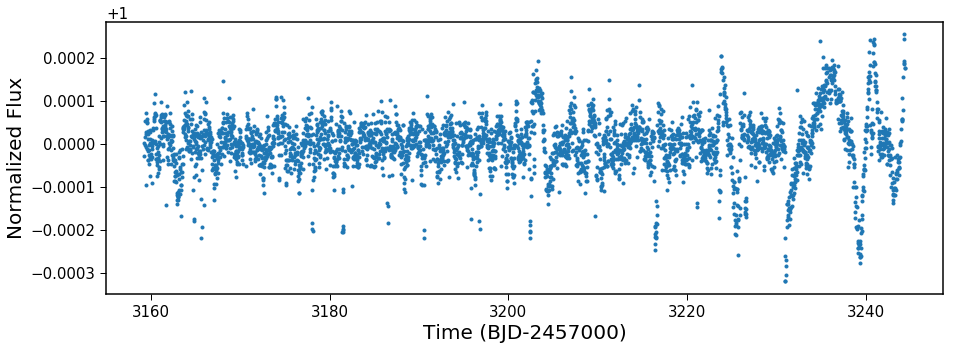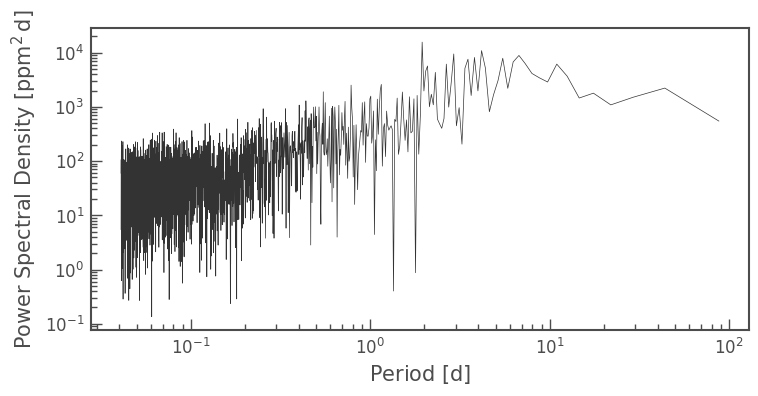The transit events are clear in the light curve, and there are some wiggles on shorter timescales too. A periodogram just picks them up at ~2 days, but it's not a particularly significant find. I wonder what that's coming from? Maybe we could try to build a light curve with a different aperture. Let's just take the pixels on the top half of the star, run those through EVEREST, and see what we get. (I did this already, the aperture is below, and I called this `top.fits` below. It's also uploaded into this directory.)

In :
```import matplotlib.image as mpimg
plt.figure(figsize=(10,10))

plt.imshow(img)
plt.xticks([])
plt.yticks([])
plt.show()
```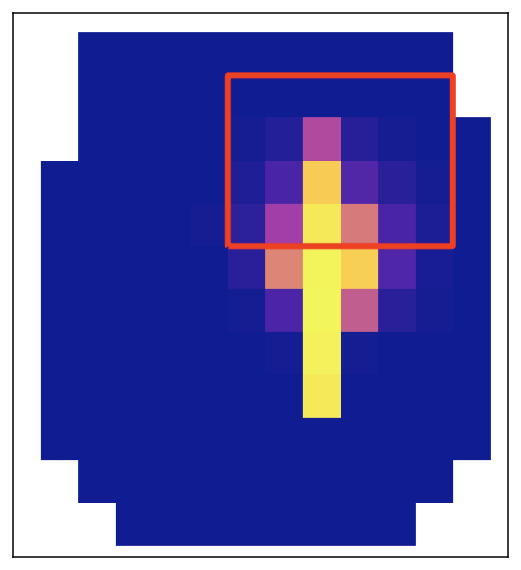Note this aperture is in the direction of the K star, just south of the primary target. Now let's make the same plots as before, with this aperture. Something jumps out immediately. The transits aren't as obvious! Where even in the non-detrended data previously we could see transit events, we don't see them. This might be because they're not there, or possibly because we have a much larger signal in this part of the aperture that is overwhelming them. We do see that wiggle more significantly though. In the detrended data, it is very clear. We can run a periodogram and see there's a very significant peak at 1.95 days.

In :
```fitsfile = 'top.fits'
a = fits.open(fitsfile)

plt.figure(figsize=(15,5))
plt.plot(a.data['time'], a.data['flux'], '.')

plt.xlabel('Time (BJD-2457000)')
plt.ylabel('Flux')

plt.show()

plt.figure(figsize=(15,5))
qual  = a.data['quality']
q = qual == 0

lk = LC(a.data['time'][q], a.data['flux'][q])

lkf = lk.flatten(window_length=201).remove_outliers()
plt.plot(lkf.time[100:], lkf.flux[100:], '.')

plt.xlabel('Time (BJD-2457000)')
plt.ylabel('Normalized Flux')

plt.show()

lkp = lkf.to_periodogram()

lkp.plot(format='period', scale='log')
peak = 1/lkp.frequency_at_max_power.value
```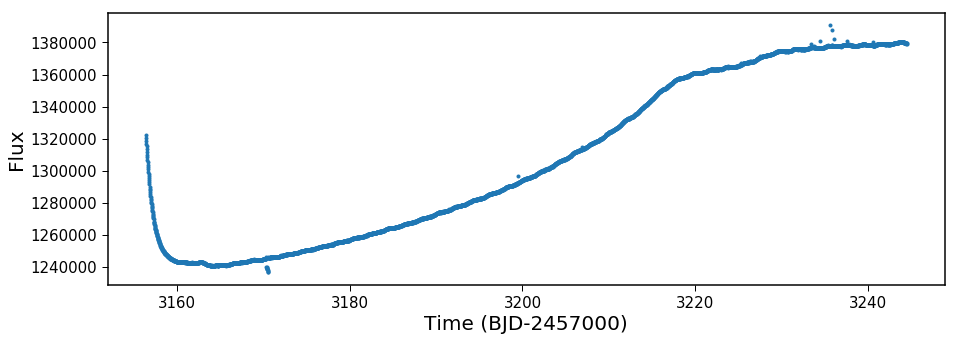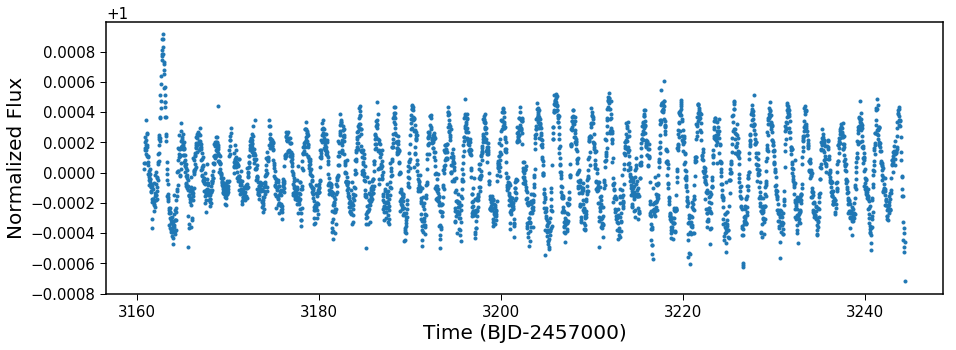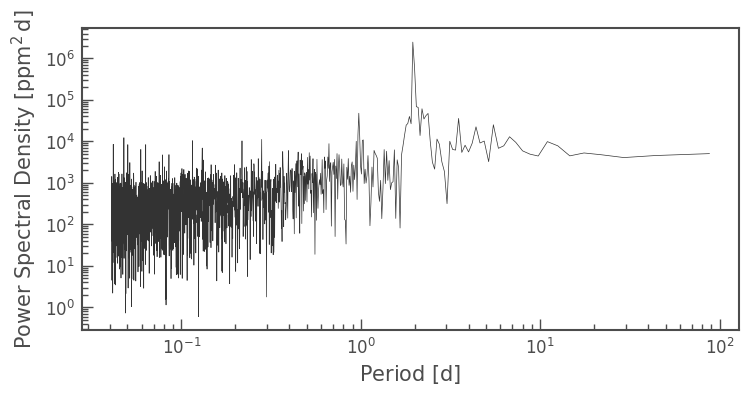Let's phase fold on that period, how does it look? Is the variability stable over the 3 months of a K2 campaign?

In :
```plt.figure(figsize=(15,5))
plt.plot(np.mod(lkf.time, peak*2)/peak, lkf.flux, '.')
plt.xlabel('Phase')
plt.ylabel('Normalized Flux')
plt.show()
```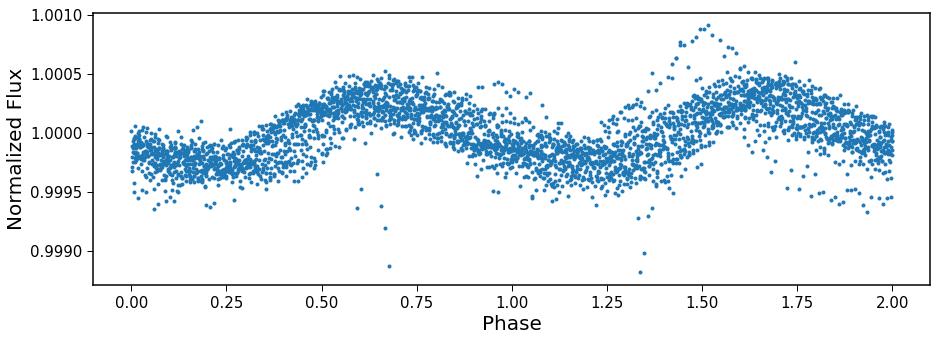Yes! It is! This looks a lot like what we'd expect from a binary star, either Doppler beaming from a star in a 2-day orbit around another star or ellipsoidal variations from a 4-day binary. In either case, there's some short period something going on in this aperture. Maybe there's a circumbinary planet? Or maybe some of these dips are an issue of detrending? For K2, a de-trended light curve is generally created by doing some flavor of decorrelation against flux values, either through the values of individual pixels or regressing against the inferred centroid in time. If there is a variable star, this would affect the centroid in time, which might affect the results of the de-trending.

Let's check to see if it's possible. Do the transits preferentially occur at some phases in this periodic variability? Let's take a single period and just plot the times of transits as vertical lines.

In :
```plt.figure(figsize=(15,5))

plt.plot(np.mod(lkf.time, peak)/peak, lkf.flux, '.')

for i in range(len(times)):
plt.axvline(np.mod(times[i], peak)/peak)
plt.xlabel('Phase')
plt.ylabel('Normalized Flux')
plt.show()
```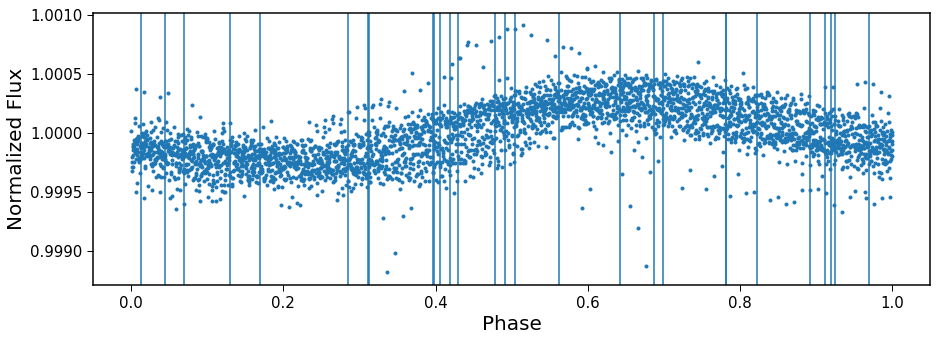Mayyyybe? There are transits all over (good!) but about a third of the transits occur at phase 0.4 or a half phase after that, 0.9. Maybe we should be a little concerned about those specific events, and we should look at them in more detail, but that's beyond the scope of this particular blog post.

Should we be cautious of any other transit events? Probably! The light curve itself from the Kepler team has had a background flux in every pixel already subtracted. If the background is temporarily overestimated, then the stellar flux would be temporarily underestimated, so a bump in the background would look like a dip in the stellar flux. A couple dips seem to have this effect. Let's zoom in on one (where I've played with the scaling to get the two light curves on the same-ish scale). Here's the background frame and the `k2sff` light curve:

In :
```plt.figure(figsize=(15,5))
plt.plot(tpf.data['time'], np.nansum(tpf.data['flux_bkg'], axis=(1,2))/44300, 'r', label='Background')
plt.plot(b.data['t'], (b.data['fcor']-1)*20+1, 'k', label='Flux')

for i in range(len(times)):
if i == 0:
plt.axvline(times[i], label='Time of transit event')
else:
plt.axvline(times[i])

plt.legend(fontsize=12)

plt.xlim(3194, 3198)
plt.ylim(0.98, 1.02)

plt.xlabel('Time (BJD-2457000)')
plt.ylabel('Normalized Flux')

plt.show()

#b.data.columns
```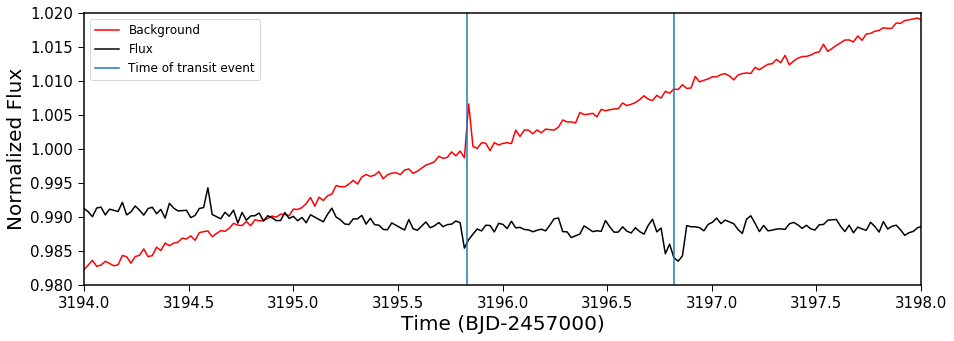The good news is that dip at 3196.8 seems secure, but the one at 3195.8 is very well aligned with a bump in the sky background, so this is probably an artifact of the data processing. In this case, the origin of these two dips are likely different: the first seems an instrumental artifact while the second, based on this simple test, looks more likely to be astrophysical.

So where does that leave us? I'm not sure. The distribution of durations and depths in the discovery paper looks a lot like what one might expect from a circumbinary planet. When I saw the short period signature pop out, I thought that might be a piece of the puzzle---these might be ellipsoidal variations on a 4-day binary, and then we have a planet going around that system, everything is sorted but the fine details. But it seems to me that the dips aren't as obvious on that half of the light curve. I don't think none of the dips are real. It's possibly they're getting lost in the noise of the periodic variability, but if they are intrinsic to this part of the aperture, then as the periodic signal grew in amplitude, they should have too. Perhaps the transits are on the primary star, or on a star on the bottom half of the aperture, so they're not caught in this EVEREST aperture. However, I'm not ready to rule out they're up here, especially since the primary is saturated, which might affect our confidence in centroiding. I'd love to see a model of a circumbinary planet around a 3.9 day period binary star that reproduces at least some of these transit times and durations though, if a physical model was built that did that I think it would be the leading candidate to explain this system!

There's a chance this star might be a combination of a few things: maybe there's a 3 planet system around the bright primary star, a few dips snuck in from bad detrending caused by the background variability from some star on the top of the detector, a few from back background modeling, and that's our story (maybe we got very unlucky, and there's also a few transits from a background star). That feels unlikely, like a 1-in-500,000 shot. Normally we should toss out super unlikely events as super unlikely, but Kepler has looked at 500,000 stars between the primary mission and K2, and so by now, 1-in-500,000 events should be observed to happen once!